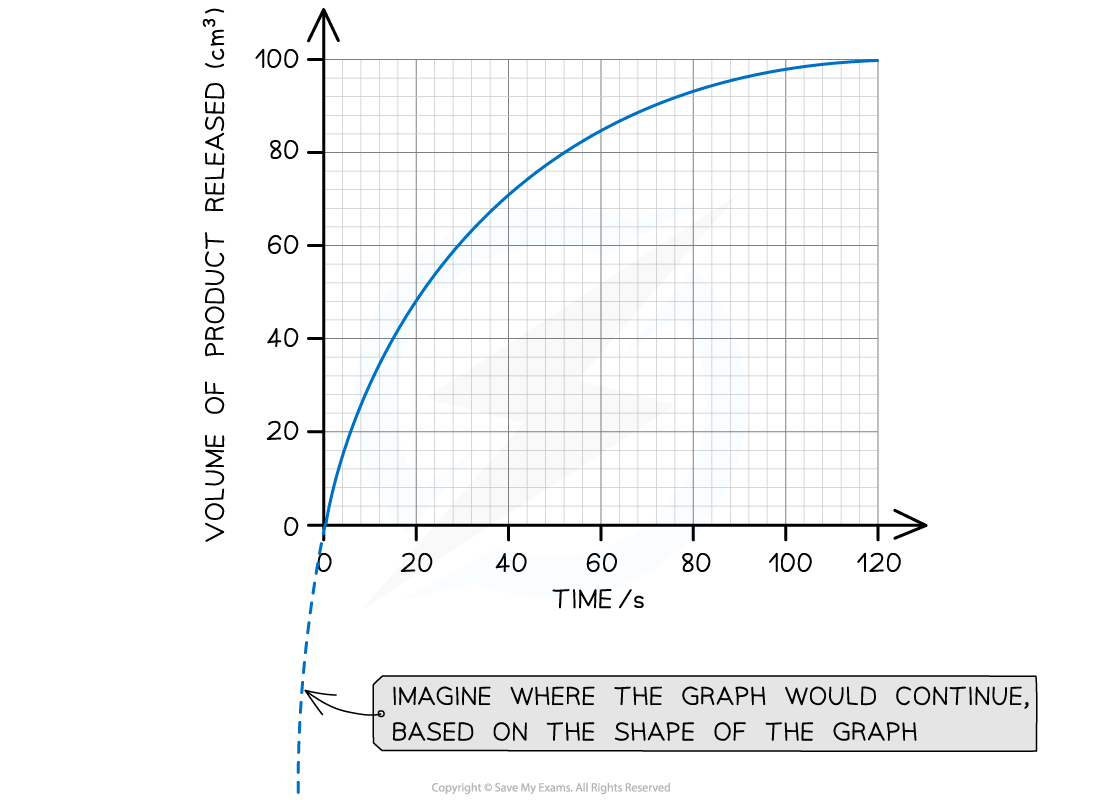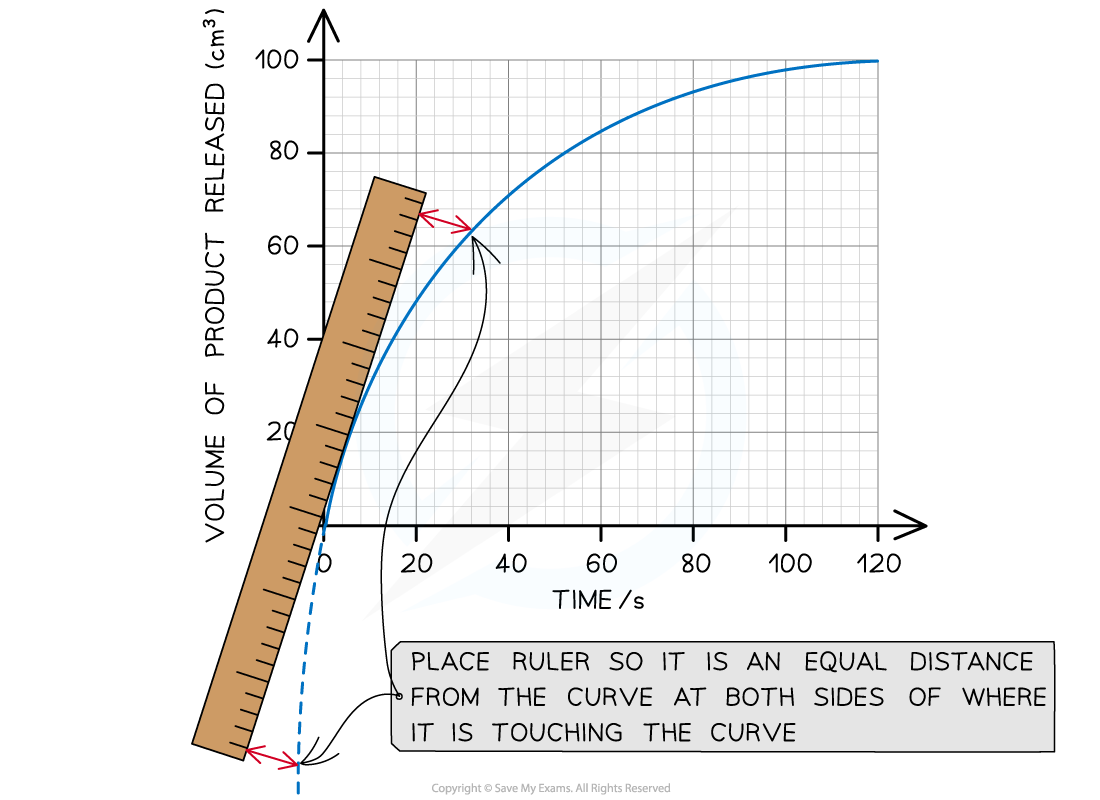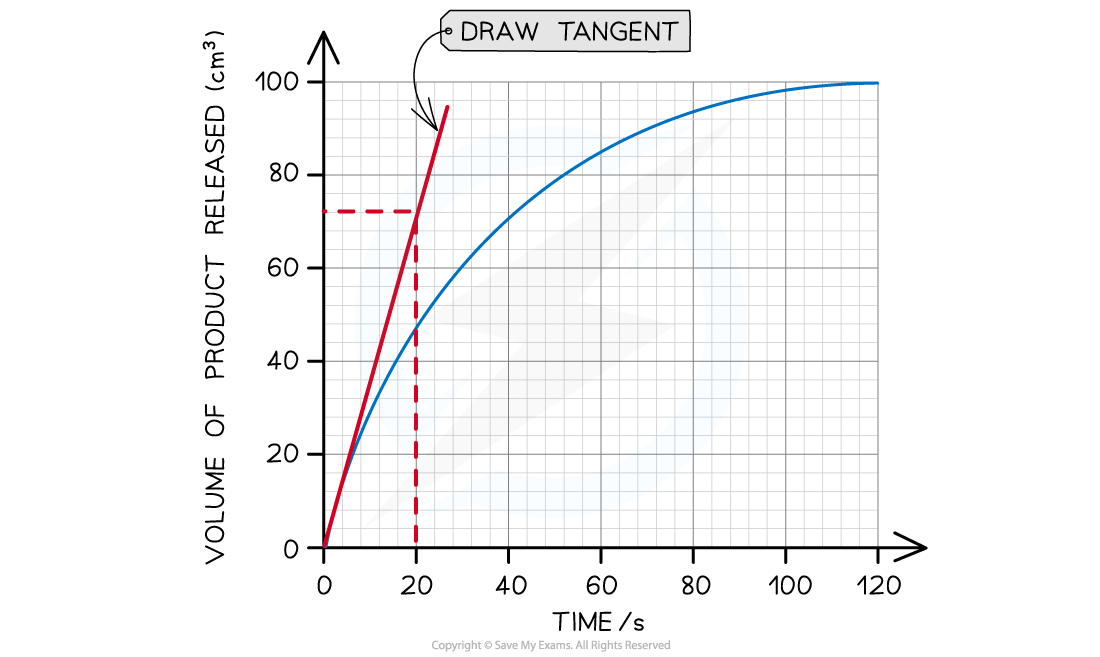# AQA A Level Biology复习笔记1.4.6 Maths Skill: Using a Tangent to Find Initial Rate of Reaction

### Maths Skill: Using a Tangent to Find Initial Rate of Reaction

• For linear graphs (i.e. graphs with a straight-line), the gradient is the same throughout
• This makes it easy to calculate the rate of change (rate of change = change ÷ time)

• However, many enzyme rate experiments produce non-linear graphs (i.e. graphs with a curved line), meaning they have an ever-changing gradient
• They are shaped this way because the reaction rate is changing over time

• In these cases, a tangent can be used to find the reaction rate at any one point on the graph:
• A tangent is a straight line that is drawn so it just touches the curve at a single point
• The slope of this tangent matches the slope of the curve at just that point
• You then simply find the gradient of the straight line (tangent) you have drawn

• The initial rate of reaction is the rate of reaction at the start of the reaction (i.e. where time = 0)

#### Worked Example

The graph below shows the results of an enzyme rate reaction. Using this graph, calculate the initial rate of reaction.Step 1: Estimate the extrapolated curve of the graphStep 2: Find the tangent to the curve at 0 seconds (the start of the reaction)The tangent drawn in the graph above shows that 72 cm3 of product was produced in the first  20 seconds.Step 3: Calculate the gradient of the tangent (this will give you the initial rate of reaction):

Gradient = change in y-axis ÷ change in x-axis

Initial rate of reaction = 72 cm3 ÷ 20 s

Initial rate of reaction = 3.6 cm3 s-1